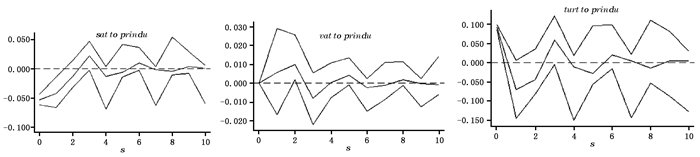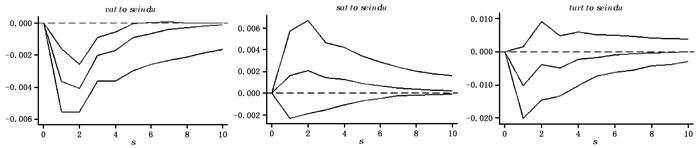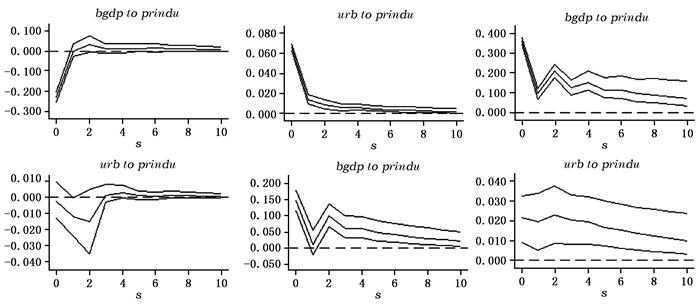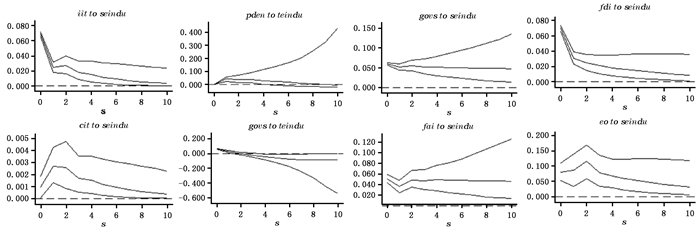﻿ 流转税改革促进了产业结构演进升级吗?--基于“营改增”视角的PVAR模型分析
 财经研究2017, Vol. 43Issue (2): 70-830

#### 文章信息财经研究2017年43卷第2期

Sun Zheng.

Does the Reform of Turnover Tax Promote the Upgrading of Industrial Structure?An Analysis of PVAR Model from a Perspective of Business-to-VAT Reform

Journal of Finance and Economics, 2017, 43(2): 70-83.

### 文章历史《财经研究》
2017第43卷第2期

Does the Reform of Turnover Tax Promote the Upgrading of Industrial Structure?An Analysis of PVAR Model from a Perspective of Business-to-VAT Reform
Sun Zheng
School of Economics, Tianjin University of Finance and Economics, Tianjin 300202, China
Abstract: Based on the non-equilibrium growth model proposed by Baumol (1967), and the exploration of a new round of fiscal and taxation reform, this paper examines the internal economic logic between the business-to-VAT reform and the evolution of industrial structure upgrading through a simple theoretical analysis.Then based on the provincial panel data from 1995 to 2014, it uses the panel vector autoregressive model to empirically analyze the influence of the business-to-VAT reform on the evolution of China's industrial structure upgrading.It arrives at the results as follows:firstly, in terms of the results of GMM and impulse response, the new round of turnover tax reform which is based on the business-to-VAT reform, improves the proportion of the tertiary industry in the national economy and reduces the proportion of the secondary industry, thereby promoting the evolution of industrial structure upgrading; secondly, in terms of the results of variance decomposition, it can be seen that the policy-shock impact of the business tax variable on the secondary industry is greater, and the policy-shock impact of the VAT variable on the tertiary industry is greater; and the effect of the turnover tax reform on the tertiary industry is greater than the one on the secondary industry.
Key words: the business-to-VAT reform    turnover tax    PVAR    industrial structure

1.行业税负效应。一方面，以“营改增”为主线索的税制改革所带来的减税效应，降低了第二产业与第三产业的总体税负，也就是说无论是制造业、服务业，还是商贸业，其税负都有所降低，有利于社会资源更多地配置到这两个产业；另一方面，“营改增”实施后，第三产业取得的增值税进项税额可以抵扣，直接降低了第三产业的行业税负，而且相对于第二产业，“营改增”对第三产业的税负降低效应更大。因此，“营改增”彻底推广到全行业以后，总体来看对第三产业税负的降低效应更明显。

2.资源配置效应。增值税具有税收中性的特点。一方面，随着二三产业之间以及第三产业内部增值税抵扣链条的完善，减少了税收对资源配置的扭曲，使资源在行业、产业之间的配置更趋合理，有利于第三产业的要素整合，提高其资源配置效率，引导资源更多地配置到第三产业；另一方面，“营改增”改变了第二产业与第三产业的相对资金成本，相对于第二产业，提高了第三产业的资本回报率，资本的逐利性决定了企业必然会进入资本回报率高的产业，最终改变企业的投资行为与生产结构，使社会资源和社会资本更多地配置到第三产业，从而促进产业结构的升级演进。

3.产业结构调整效应。营业税具有重复征税的弊端，“营改增”彻底避免了对服务业的重复征税，消除了第三产业发展的税收制度性障碍，有利于产业之间分工细化与产业之间的融合发展，产业发展也从以产品为中心逐渐转变为以服务为中心，推动产业结构服务化，进而提升产业层次。特别是以“营改增”为主线索的流转税改革所形成的下游企业增值税进项税抵扣与企业本身进项税抵扣的双重效应，对第三产业发展壮大的政策支持效应明显。另外，“营改增”改革以后，第二产业购买服务产品，尤其是基础研发、技术咨询等生产性服务，可以相应抵扣一部分进项税，有利于传统工业向服务型工业转型。

(二) 简单理论模型构建。

 $seind{u_{it}} = {\left( {\rho {L_{it}}} \right)^\alpha }K_{it}^\beta G_{it}^\gamma$ (1)
 $teind{u_{it}} = {\left[ {\left( {1 - \rho } \right){L_{it}}} \right]^\sigma }seindu_{it}^\varepsilon$ (2)
 ${{\dot K}_{it}} = \kappa seind{u_{it}}$ (3)
 ${{\dot L}_{it}} = n{L_{it}}$ (4)
 ${{\dot G}_{it}} = g{G_{it}}$ (5)
 ${{\dot G}_{it}} = g{G_{it}}$ (6)

 ${\pi _\kappa } = \dot K/K = \kappa seind{u_{it}}/K = \kappa {\left( {\rho {L_{it}}} \right)^\alpha }K_{it}^{\beta - 1}G_{it}^\gamma$ (7)

 ${{\dot \pi }_\kappa } = \alpha n + \left( {\beta - 1} \right){\pi _\kappa } + \gamma g$ (8)

 ${\pi _\kappa } = \left( {\alpha n + \gamma g} \right)/\left( {1 - \beta } \right)$ (9)

 ${\pi _{seindu}} = \alpha n + \beta {\pi _\kappa } + \gamma g = \left( {\alpha n + \gamma g} \right)/\left( {1 - \beta } \right)$ (10)

 ${\pi _{teindu}} = \rho n + \varepsilon {\pi _{seindu}} = \left[ {\left( {1 - \beta } \right)\rho n + \alpha \varepsilon n + \gamma \varepsilon g} \right]/\left( {1 - \beta } \right)$ (11)

“营改增”彻底推广到全行业，增值税取代营业税，也就意味着营业税、增值税并行于第二产业与第三产业的流转税二元税制模式转化为一元税制模式，“营改增”从本质上说是第三产业税负的降低，有利于资源配置倾向于第三产业，促进传统工业服务化。为了更好地考察“营改增”对产业结构升级的影响，本文令λ表示第三产业产出增长率与第二产业产出增长率的比值，即：λ=πteindu/πseindu，如果“营改增”带来第三产业的增长速度快于第二产业的增长速度，那么我们就认为“营改增”促进了产业结构的演进升级，可以得到：

 $\lambda = {\pi _{teindu}}/{\pi _{seindu}} = [(1 - \beta )\rho n + \alpha \varepsilon n + \gamma \varepsilon g]/(\alpha n + \gamma g) = (1 - \beta )\rho n/(\alpha n + \gamma g) + \varepsilon$ (12)

 $\lambda = (1 - \beta )\rho n/(\alpha n + \gamma \tau ) + \varepsilon$ (13)

 $\lambda = (1 - \beta )\rho n/[\alpha n + f(taxsys)\gamma ] + \varepsilon$ (14)

 $\partial \lambda /\partial taxsys = - \{ (1 - \beta )\gamma \rho n/{[\alpha n + f(taxsys)\gamma ]^2}\} f'(taxsys)$ (15)
 $\partial \lambda /\partial taxsys > 0$ (16)

(一) 计量模型构建。

 ${Z_{it}} = {\prod _0} + \sum\limits_{p = 1}^n {{\prod _{np}}} {Z_{it{\rm{ - }}p}} + \sum\limits_{p = 1}^n {{\coprod _{np}}} {X_{it{\rm{ - }}p}} + {\varphi _t}{f_i} + {\varepsilon _i} + {\mu _{it}}$ (17)

 $prind{u_{it}} = {\prod _0} + \sum\limits_{p = 1}^n {{\prod _{np}}} prind{u_{it{\rm{ - }}p}} + \sum\limits_{p = 1}^n {{\coprod _{np}}} ta{x_{it{\rm{ - }}p}} + \sum\limits_{p = 1}^n {{\coprod _{np}}} {X_{it{\rm{ - }}p}} + {\varphi _t}{f_i} + {\varepsilon _i} + {\mu _{it}}$ (18)
 $seind{u_{it}} = {\prod _0} + \sum\limits_{p = 1}^n {{\prod _{np}}} seind{u_{it{\rm{ - }}p}} + \sum\limits_{p = 1}^n {{\coprod _{np}}} ta{x_{it{\rm{ - }}p}} + \sum\limits_{p = 1}^n {{\coprod _{np}}} {X_{it{\rm{ - }}p}} + {\varphi _t}{f_i} + {\varepsilon _i} + {\mu _{it}}$ (19)
 $teind{u_{it}} = {\prod _0} + \sum\limits_{p = 1}^n {{\prod _{np}}} teind{u_{it{\rm{ - }}p}} + \sum\limits_{p = 1}^n {{\coprod _{np}}} ta{x_{it{\rm{ - }}p}} + \sum\limits_{p = 1}^n {{\coprod _{np}}} {X_{it{\rm{ - }}p}} + {\varphi _t}{f_i} + {\varepsilon _i} + {\mu _{it}}$ (20)

(二) 变量与数据。

1.数据来源。样本选取30个省份的数据，样本区间为1995-2014年，共20个年度的数据组。由于重庆1997年设直辖市，缺少1995年和1996年数据，所以本文面板数据为非平衡面板。产业结构数据主要来源于1996-2015年《中国统计年鉴》以及30个省级单位的统计年鉴，流转税、营业税和增值税等税收相关数据来源于《中国财政年鉴》和《中国税收年鉴》，控制变量组数据主要通过《中国统计年鉴》《中国对外经贸年鉴》《中国区域经济年鉴》《中国人口统计年鉴》以及wind数据库等获取。

2.变量设定与数据的描述性统计。被解释变量：主要包括国民经济中第一产业、第二产业和第三产业的占比。其中，(prinduit)为国民经济中第一产业占GDP比重，prinduit-1为上一期第一产业比重；(seinduit)为国民经济中第二产业占GDP比重，seinduit-1为上一期第二产业比重；(teinduit)为国民经济中第三产业占GDP比重，teinduit-1为上一期第三产业比重。

 变量名称 变量定义 被解释变量 第一产业(prinduit) 各省级单位第一产业占GDP的比重 第二产业(seinduit) 各省级单位第二产业占GDP的比重 第三产业(teinduit) 各省级单位第二产业占GDP的比重 核心解释变量 营业税(satit) 各省级单位营业税占GDP的比重 增值税(vatit) 各省级单位增值税占GDP的比重 流转税(turtit) 各省级单位流转税占GDP的比重，包括营业税、增值税、消费税 企业所得税(citit) 各省级单位企业所得税占GDP的比重 个人所得税(iitit) 各省级单位个人所得税占GDP的比重 控制变量 固定资产投资比率(faiit) 各省级单位固定投资总额占GDP的比重 城镇化进程(urbit) 各省级单位城镇人口占总人口的比重 利用外资水平(fdiit) 各省级单位利用外资规模占GDP的比重 经济开放度(eoit) 各省级单位进出口总额占GDP的比重 政府规模(govsit) 各省级单位“消费”占GDP的比重 经济发展程度(pgdpit) 各省级单位人均GDP的自然对数值 人口密度(pdenit) 各省级单位总人口/各省份面积 注：变量为作者定义。

 变量名 样本数 均值 标准差 最小值 最大值 prinduit 600 0.1570547 0.0939785 0.0052725 0.503 seinduit 600 0.455774 0.0792246 0.198 0.615 teinduit 600 0.3871976 0.0868286 0.1160329 0.779484 satit 600 0.0221492 0.0136106 0 0.1009 vatit 600 0.0628419 0.0318798 0 0.2308033 turtit 600 0.0974808 0.0453191 0.005662 0.3235651 citit 600 0.024505 0.0321469 0.0038658 0.270887 iitit 600 0.0081713 0.0070993 0 0.0544605 faiit 600 0.4376163 0.2161931 0 1.210822 urbit 600 0.4213402 0.2057419 0 0.896 fdiit 600 0.0658751 0.0849232 0 0.7503114 eoit 600 0.3064793 0.3887334 0.0316411 1.817133 govsit 600 0.1650383 0.0833134 0 0.6274226 pdenit 600 5.389442 1.25479 2.00986 8.255902 pgdpit 600 9.549007 0.9070899 1.896012 8.255902 资料来源：见前述“数据来源”部分的阐述。

(一) 平稳性检验。

 检验方法 prinduit seinduit teinduit stit vatit LLC -12.25*** -3.50*** -7.75*** -3.53*** -10.48*** IPS -11.56*** -12.99*** -14.87*** -16.71*** -12.25*** HT -32.08*** -35.51*** -34.20*** -36.39*** -36.62*** 检验方法 turtit citit iitit urbit pdenit LLC -11.66*** -2.26** -7.03*** -12.00*** -1.72* IPS -12.86*** -18.89*** -15.99*** -12.32*** -6.31*** HT -36.49*** -35.07*** -38.01*** -36.23*** -19.65*** 检验方法 faiit eoit fdiit govsit pgdpit LLC -3.03** -10.94*** -9.46*** -3.06** -8.43*** IPS -10.01*** -13.18*** -12.42*** -5.16*** -10.15*** HT -28.06*** -32.85*** -40.54*** -13.76*** -24.94*** 注：*、**和***分别表示该系数在10%、5%和1%的置信水平通过检验。表 4同。
(二) 面板向量自回归(PVAR)的参数估计。

(三) 主要变量实证结果分析。

1.第一产业。由传统VAR模型的原理可知，面板向量自回归模型并不区分内生变量与外生变量，而是将所有的变量都等同为内生变量。所以这里把产业结构变量、营业税、增值税和流转税等均作为PVAR模型的内生变量。进行“营改增”改革对第一产业占比政策冲击的检验，主要来自式(18)的实证分析结果。由表 4估计结果可见，被解释变量为h_prindu方程中，滞后一期与滞后二期的h_sath_vat变量的实证检验结果都不显著，“营改增”对第一产业的占比变动没有影响，或是解释力度不大，这主要是由于流转税以第二产业与第三产业为课税主体。另外，滞后一期与滞后二期变量h_prindu系数都为正，这说明第一产业所占比重存在着自我增强机制。通过对比，由被解释变量为h_sath_vat的方程可见，第一产业、营业税和增值税变量之间PVAR模型的GMM估计结果不显著，这也从侧面说明“营改增”对第一产业变动没有影响。

 h_prindu方程 h_sat方程 h_vat方程 变量 系数 变量 系数 变量 系数 h_prindu(-1) -1.33*(-1.79) h_prindu(-1) -0.68(-0.51) h_prindu(-1) -0.76(-0.51) h_prindu(-2) -1.21**(2.14) h_prindu(-2) -0.59(-0.5) h_prindu(-2) -0.58(-0.44) h_sat(-1) 0.42*(1.72) h_st(-1) -0.52(-0.37) h_st(-1) -1.83(-1.19) h_sat(-2) 0.13(0.53) h_st(-2) -0.80(-0.57) h_st(-2) -1.43(-0.84) h_vat(-1) -3.75(-0.55) h_vat(-1) -1.69(-0.52) h_vat(-1) -1.46(-0.41) h_vat(-2) -10.09(-0.56) h_vat(-2) -1.51(-0.49) h_vat(-2) -1.33(-0.39) 注：使用stata12软件整理，h表示对各个变量进行前向差分，括号内数字为估计系数的t检验值。图 1 “营改增”对第一产业的冲击(蒙特卡罗模拟500次)

 被冲击变量 期数 冲击变量 prinduit stit vatit prinduit 1 1.000 0.000 0.000 stit 1 0.070 0.930 0.000 vatit 1 0.044 0.190 0.766 prinduit 5 0.960 0.020 0.020 stit 5 0.020 0.960 0.020 vatit 5 0.960 0.020 0.020 prinduit 10 0.020 0.960 0.020 stit 10 0.020 0.960 0.020 vatit 10 0.020 0.960 0.020

2.第二产业。对“营改增”改革影响第二产业占比政策冲击效果的检验，主要来自式(19)的实证分析结果。这里同样通过PVAR模型的GMM估计、脉冲响应图和方差分解三个层面考察“营改增”对第二产业占比的政策冲击。

 h_seindu方程 h_sat方程 h_vat方程 变量 系数 变量 系数 变量 系数 h_seindu(-1) 1.05***(5.4) h_seindu(-1) 0.89**(2.46) h_seindu(-1) -1.11**(2.35) h_seindu(-2) 0.93**(2.46) h_seindu(-2) 0.68*(1.73) h_seindu(-2) -0.86**(-1.82) h_sat(-1) 1.50**(2.33) h_sat(-1) -11.84*(2.13) h_sat(-1) -15.93(-1.47) h_sat(-2) 0.86*(1.78) h_sat(-2) -3.52(0.92) h_sat(-2) -4.95(-0.50) h_vat(-1) -2.40**(-2.42) h_vat(-1) 2.27(0.76) h_vat(-1) 3.13(1.49) h_vat(-2) -1.02**(2.26) h_vat(-2) 0.84(0.88) h_vat(-2) 1.29(0.71)图 2 “营改增”对第二产业的冲击(蒙特卡罗模拟500次)

 被冲击变量 期数 冲击变量 seinduit stit vatit seinduit 1 0.891 0.0397 0.0693 stit 1 0.095 0.8493 0.0557 vatit 1 0.1083 0.1682 0.7235 seinduit 5 0.9017 0.0348 0.0635 stit 5 0.1956 0.7026 0.1018 vatit 5 0.0758 0.135 0.7892 seinduit 10 0.9083 0.0308 0.0609 stit 10 0.2233 0.6592 0.1175 vatit 10 0.1734 0.1245 0.7021

3.第三产业。“营改增”改革对第三产业占比政策冲击的检验，主要来自式(20)的实证分析结果。这里同样通过PVAR模型的GMM估计、脉冲响应图和方差分解三个层次考察“营改增”对第三产业占比的政策冲击。

 h_teindu方程 h_sat方程 h_vat方程 变量 系数 变量 系数 变量 系数 h_teindu(-1) 0.35***(5.18) h_teindu(-1) -0.12**(-3.62) h_teindu(-1) 0.08*(1.85) h_teindu(-2) -0.15(-0.93) h_teindu(-2) -0.11***(-3.22) h_teindu(-2) 0.21***(3.17) h_sat(-1) -2.25**(-2.08) h_sat(-1) 0.41**(2.54) h_sat(-1) -0.85(1.69) h_sat(-2) -0.41*(-1.48) h_sat(-2) 0.47(2.73) h_sat(-2) 0.42(1.15) h_vat(-1) 1.34*(1.85) h_vat(-1) 0.05(1.26) h_vat(-1) 0.34***(5.10) h_vat(-2) 0.26***(3.47) h_vat(-2) 0.04**(2.08) h_vat(-2) 0.31***(8.91)图 3 “营改增”对第三产业的冲击(蒙特卡罗模拟500次)

 被冲击变量 期数 冲击变量 teinduit stit vatit teinduit 1 0.8089 0.0533 0.1378 stit 1 0.0433 0.7044 0.2523 vatit 1 0.0648 0.0163 0.9189 teinduit 5 0.7982 0.0453 0.1565 stit 5 0.3214 0.5419 0.1367 vatit 5 0.1183 0.0288 0.8529 teinduit 10 0.8523 0.0428 0.1149 stit 10 0.2896 0.6062 0.1042 vatit 10 0.0609 0.1144 0.8247
(四) 控制传导变量实证结果。图 4 其他变量对产业结构升级演进的冲击(蒙特卡罗模拟500次)图 5 其他变量对产业结构升级演进的冲击(蒙特卡罗模拟500次)

(五) 稳健性估计。

  褚敏, 靳涛. 为什么中国产业结构升级步履迟缓--基于地方政府行为与国有企业垄断双重影响的探究[J]. 财贸经济, 2013(3): 112–122.  国亮, 王一笑. 土地财政对我国产业结构升级的影响--基于产业间税种差异和土地财政的视角[J]. 江西社会科学, 2015(8): 33–40.  郭小东, 刘长生, 简玉峰. 政府支出规模、要素积累与产业结构效应[J]. 南方经济, 2009(3): 51–61.  李勇刚, 王猛. 土地财政与产业结构服务化--一个解释产业结构服务化"中国悖论"的新视角[J]. 财经研究, 2015(9): 29–41.  柳光强, 田文宠. 完善促进战略性新兴产业发展的税收政策设想--从区域税收优惠到产业优惠[J]. 中央财经大学学报, 2012(3): 1–5.  石奇, 孔群喜. 动态效率、生产性公共支出与结构效应[J]. 经济研究, 2012(1): 92–104.  王文举, 向其凤. 中国产业结构调整及其节能减排潜力评估[J]. 中国工业经济, 2014(1): 44–56.  魏福成, 邹薇, 马文涛, 等. 税收、价格操控与产业升级的障碍--兼论中国式财政分权的代价[J]. 经济学(季刊), 2013(7): 1491–1512.  Alvarez-Cuadrado F, Poschke M. Structural change out of agriculture:Labor push versus labor pull[J]. American Economic Journal:Macroeconomics, 2011, 3(3): 127–158. DOI:10.1257/mac.3.3.127  Buera F J, Kaboski J. The rise of the service economy[J]. The American Economic Review, 2012, 102(6): 2540–2569. DOI:10.1257/aer.102.6.2540  Herrendorf B, Rogerson R, Valentinyi A. Two perspectives on preferences and structural transformation[J]. The American Economic Review, 2013, 103(7): 2752–2789. DOI:10.1257/aer.103.7.2752  Rogerson R.Structural transformation and the deterioration of European labor market outcomes[R].National Bureau of Economic Research, 2008, 116(2):235-259.